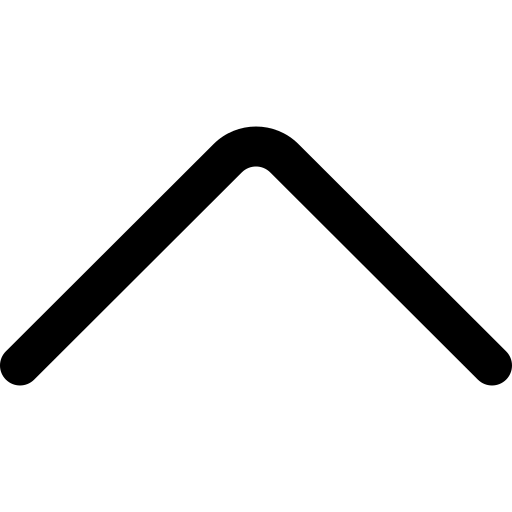HomeQuantitative TechniquesMaths: Number System for CLAT

# Maths: Number System for CLAT

#### NUMBER SYSTEM

Number system comprises of 10 digits (0, 1, 2,3,4,5,6,7,8,9 ).  When these digits are grouped together it becomes a number. The place at which the digits are placed is its Place Value. But the value of digit remains the same wherever it is placed hence we call it as Face Value

Ex. Let us take a number 395284 and place the digits in place value chart.

 Place Value Lacs Ten Thousand Thousands Hundreds Tens Units Digit 3 9 5 2 8 4 Power of 10 5 4 3 2 1 0

Types of Numbers:

1. Natural Numbers: Positive numbers (1,2,3,4,5,6……) beginning from 1 that can be counted are known as Natural Numbers. These numbers are also known as Positive Integers.
2. Whole Numbers: All the numbers that can be counted including zero ( 0,1,2,3,4….) are known as Whole Numbers.
3. Integers: All the numbers that can be counted (positive and negative) and 0 are Integers. …-3,-2,-1,0,1,2,3….
• Positive Integers: Positive numbers including zero 0,1,2,….
• Negative Integers: Negative numbers ….-4,-3,-2,-1
1. Rational Number: Number that can be expressed in the format of (a/b) where b≠0 is a rational number. This includes all integers, zero or fraction.
2. Irrational Numbers: Numbers that cannot be expressed in proper (a/b) format are irrational numbers.
For eg: π = 1415926535897932384626433832795028… And this value of pi never terminates and we use 3.14 or 22/7 (where 22/7 is an irrational number). That’s why irrational numbers are also known for their recurring property.
3. Even Numbers: Integers (+ve & -ve) that can be divided by 2 are even numbers
4. Odd Numbers: Integers that cannot be divided by 2 are odd numbers
5. Prime Numbers: Positive Integers that have only two factors 1 and itself are Prime numbers. It is advisable to know all 25 prime numbers below 100 i.e., 2, 3, 5, 7, 11, 13, 17, 19, 23, 29, 31, 37, 41, 43, 47, 53, 59, 61, 67, 71, 73, 79, 83, 89 & 97.

NOTE: 2 is the only even prime number.

1. Composite Number: All the numbers that are not prime are Composite Numbers.
2. Co- Prime Numbers: Two numbers that have HCF as 1 i.e, no other common factor exists between them are called Co- Prime Numbers. Ex: 5 and 24
3. Real Number: All the numbers discussed above comes under the umbrella of Real numbers. These numbers can be represented in the number line.

#### Divisibility Tests

Any number is considered divisible when it is divided by other number or digit without leaving a remainder.

Divisibility Rules

• for 2: If the unit digit is 0,2,4,6,8 then that number is divisible by 2.
• for 3: A number is only divisible by 3 when the sum of all the digits of the number is divisible by 3.

Eg: 942, In the number 942351 sum of digits is  24 which is divisible by 3, therefore 942351 is also divisible by 3.

• for 4: If the last digit of a number is 0 or the Last two digits are divisible by 4 then that number is divisible by 4.
• for 5: If the number has 0 or 5 at its units place that it is divisible by 5
• for 6: If a number is divisible by 2 and 3 both are also divisible by 6
• for 7: Let’s learn this using an example 161. The first step would be to double the number at units place i.e., 1*2 = 2. Now subtract this from remaining number 16-2=14. Check the reduced number is divisible by 7 or not. If Yes than number 161 is also divisible.
• for 8: A number is only divisible by 8 when its last 3 digits (i.e., digits at hundreds, tens and units place) are divisible by 8 or if the last 3 digits are zero.

Eg: Let’s check if 1111128 is divisible by 8 or not. The last 3 digits are 128 when we check the number after dividing it to 8 it leaves no remainder, therefore we can say 1111128 is divisible by 8.

• for 9: A number is only divisible by 9 if the sum of all its digits is divisible by 9.

Ex: 652491, the sum of all the digits is 27, which is divisible by 9 therefore 652491 is also divisible by 9.

• for 11: If the difference between the sum of digits at odd places and the sum of digits at even places is either 0 or multiple of 11, then the number is divisible by 11.

Ex: 95667, sum of digits at odd places = 7+6+9 = 22;
sum of digits at even places = 5+6 = 11;
Difference = 22-11 = 11;
Number is divisible by 11 as the difference is a multiple of 11.

• for 25: If the last two digits of a number are either of these: 00, 25, 50 or 75 (i.e., multiple of 25), then the number is divisible by 25.
##### Nishi MallikaThese courses are starting soon